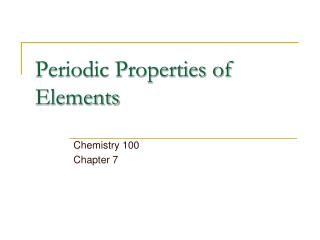Download PresentationPeriodic Properties of Elements

# Periodic Properties of Elements

Download Presentation## Periodic Properties of Elements

- - - - - - - - - - - - - - - - - - - - - - - - - - - E N D - - - - - - - - - - - - - - - - - - - - - - - - - - -
##### Presentation Transcript

1. Periodic Properties of Elements Chemistry 100 Chapter 7

2. The Modern Periodic Table

3. Atomic size • Atoms do not have a well defined size. • As the distance from the nucleus increases, it becomes less probable that an electron will be found there. • Examine a molecule of A2 • the distance between one nucleus and the other is d, then the radius of an A atom is ½d

4. An “Atomic Size” Calculation

5. Atomic radii • The C-C bond in diamond is 1.54Å, so we assign 0.77Å as the radius of the carbon atom. • The bond in Cl2 is 1.99Å long, so we give the Cl atom a radius of 0.99Å. • We predict that the C-Cl bond should be 0.77 + 0.99 = 1.76Å long. Experimental result is 1.77Å.

6. Atomic Radii and Periodic Table • As you descend a group, the atoms get larger. • This seems to be intuitive - the atoms lower in a group have more electrons and these fill higher shells. • As you cross a row, radius decreases. • The electrons are in the same shell but the nuclear charge increases as you cross a group - electrons attracted to centre.

7. Ionization energy • The first ionization energy I1, is the energy required to remove one electron from the neutral atom. • Example Na (g)  Na+ (g) + e- • The second ionization energy I2, is the energy required to remove the second electron. • Example Na+(g)  Na2+ (g) + e-

8. IE (Cont’d) • The greater the value of I, the more difficult it is to remove an electron • The first electron is more readily removed than the second, etc. I1 <I2 < I 3 < I4

9. Na [Ne]3s1 Si [Ne]3s23p2 Cl [Ne]3s23p5 Mg [Ne]3s2 P [Ne]3s23p3 Ar [Ne]3s23p6 = [Ar] Al [Ne]3s23p1 S [Ne]3s23p4 1) More difficult to remove electron from smaller atom 2) I1 <I2 < I 3 < I4 First electron easiest to remove 3) Inner-shell electrons “impossible” to remove

10. Electron Affinity • Ionization energy measures the energy change associated with the removal of an electron. Cl (g)  Cl+(g) + e- E = 1251 kJ/mol • Positive value means energy must be added to atom to remove electron • Electron Affinity measures the energy change related to the addition of an electron Cl (g) + e- Cl-(g) E = -349 kJ/mol

11. Electron Affinity (cont) • The Cl- ion is more stable than the Cl atom • Cl configuration [Ne]3s23p5 • Cl- configuration [Ne]3s23p6 • The ion has the same electron configuration as Ar - a closed shell • The Cl- ion is readily formed

12. Electron Affinity Values

13. Metals, Non-metals & Metalloids • Elements which ionize (lose electrons) readily are metals: Sodium, Iron, Lead • Elements which readily gain electrons are non-metals: Chlorine, Sulphur, Argon • Separating them are the metalloids: Boron, Silicon, Arsenic

14. Metals v Non-metals • Shiny luster, often silvery No luster, many colours • Solids are malleable (can be shaped with hammer) and ductile (can be drawn into wires) Solids often brittle; some are hard, some soft

15. Metals vs. Nonmetals (Round 2) • Good conductors of heat and electricity Poor conductors (graphite is an exception) • Most metal oxides are basic Most non-metallic oxides are acidic • Tend to form cations (+ve charge) in solution Tend to form anions or oxyanions in solutions

16. Metals • All but Hg are solids are 25ºC. (What is the other liquid element?) • Low ionization energies; form positive ions • Oxides are basic CaO(s) + H2O(l)  Ca(OH)2 (aq) Metal oxide + acid  salt + water MgO(s) + 2HCl(aq)  MgCl2(aq) + H2O(l)

17. Non-metals • Vary greatly in appearance. • Seven exist as diatomic atoms. • H2 (colourless gas) • F2 (yellowish gas) • Cl2 (green gas) • Br2 (red liquid) • I2 (purple volatile solid) • Diamond (C) is hard, sulphur is soft.

18. Nonmetals (Round 2) • Tend to gain electrons to form anions • Oxides are acidic non-metal oxide + water  acid CO2 + H2O  H2CO3 (aq) non-metal oxide + acid  salt + water SO3 + 2KOH  K2SO4 (aq) + H2O(l)

19. Aluminum Al2O3 amphoteric oxide (can act as either an acid or a base). Al2O3(s) + 6 HCl (aq)  2 AlCl3 (aq) + 3 H2O (l) (basic) Al2O3 (s) + 2 NaOH (aq) + 3 H2O (l)  2 NaAl(OH)4 (acidic oxide)

20. Metalloids • Generally hard, non-malleable solids • In pure state they are non-conductors but with controlled impurities they form semi-conductors • Computer chips are made of Si

21. Allotropy • Carbon can exist as carbon black (soot), graphite, buckyballs, or diamond. • These are called allotropes - same element, different physical appearances. • Carbon is said to exhibit allotropy

22. Allotropy (Cont’d) • Tin is a metal at 25ºC. Below 13ºC it can turn into a white, non-metallic powder. • At extremely high pressures, there is a metallic form of hydrogen.